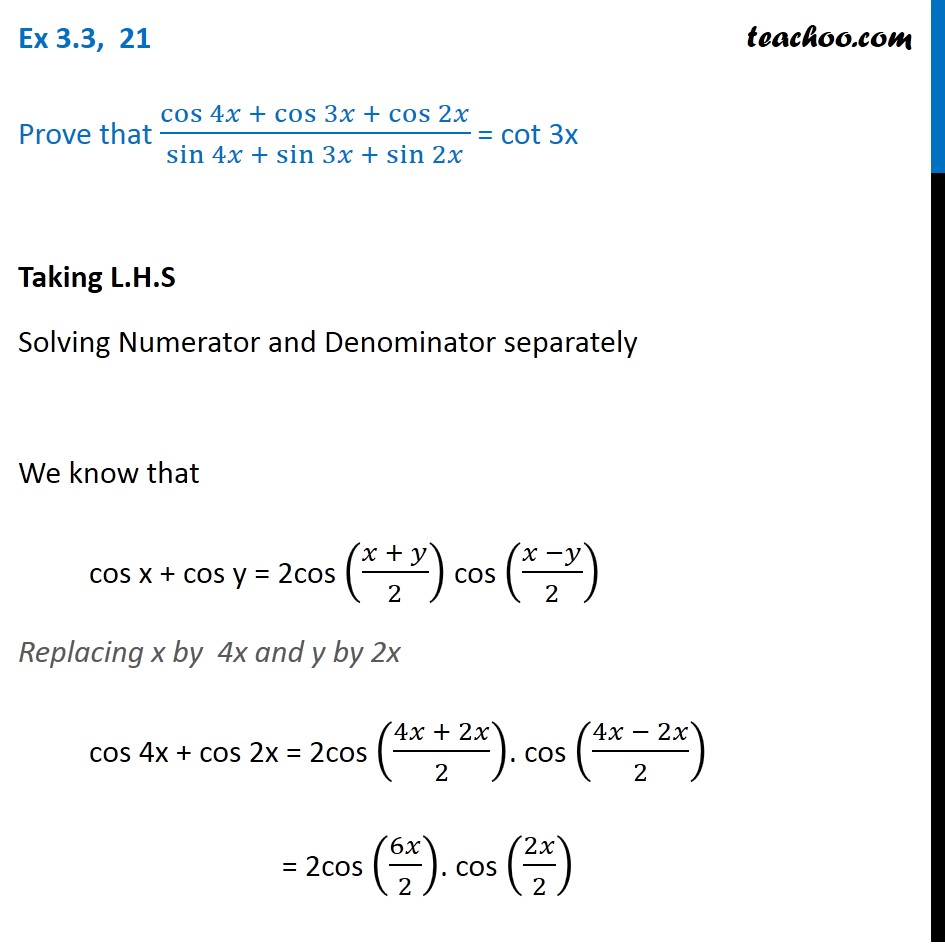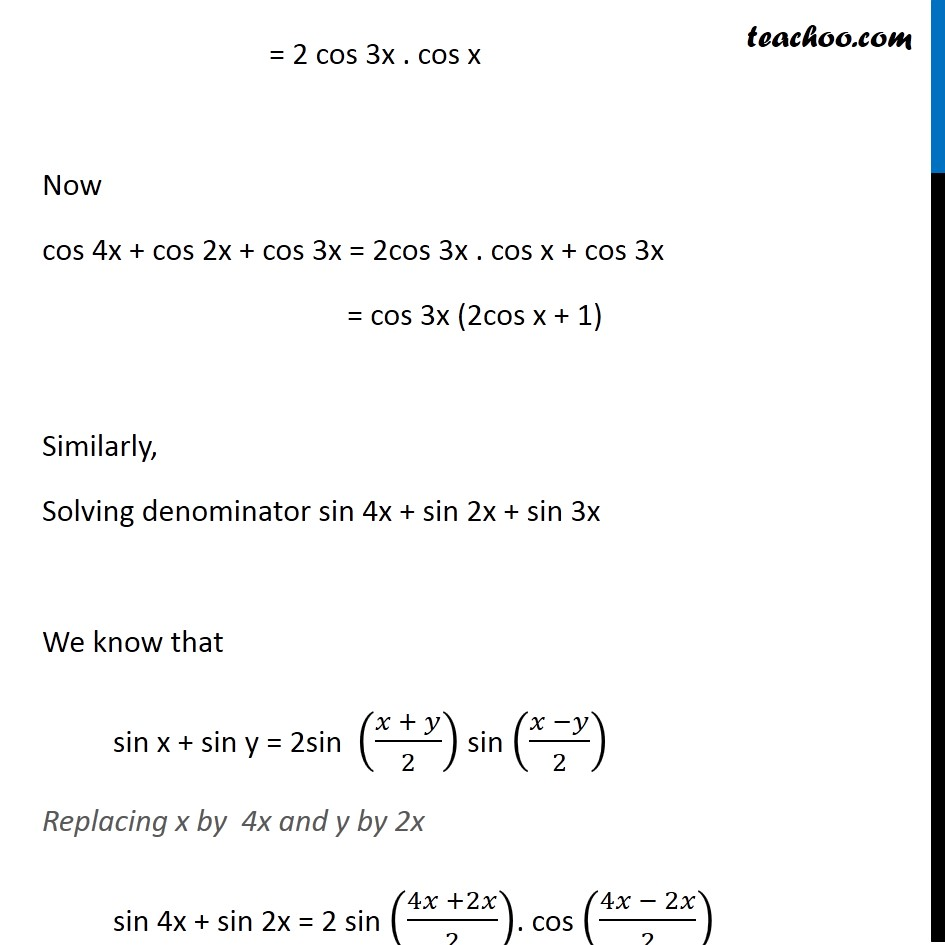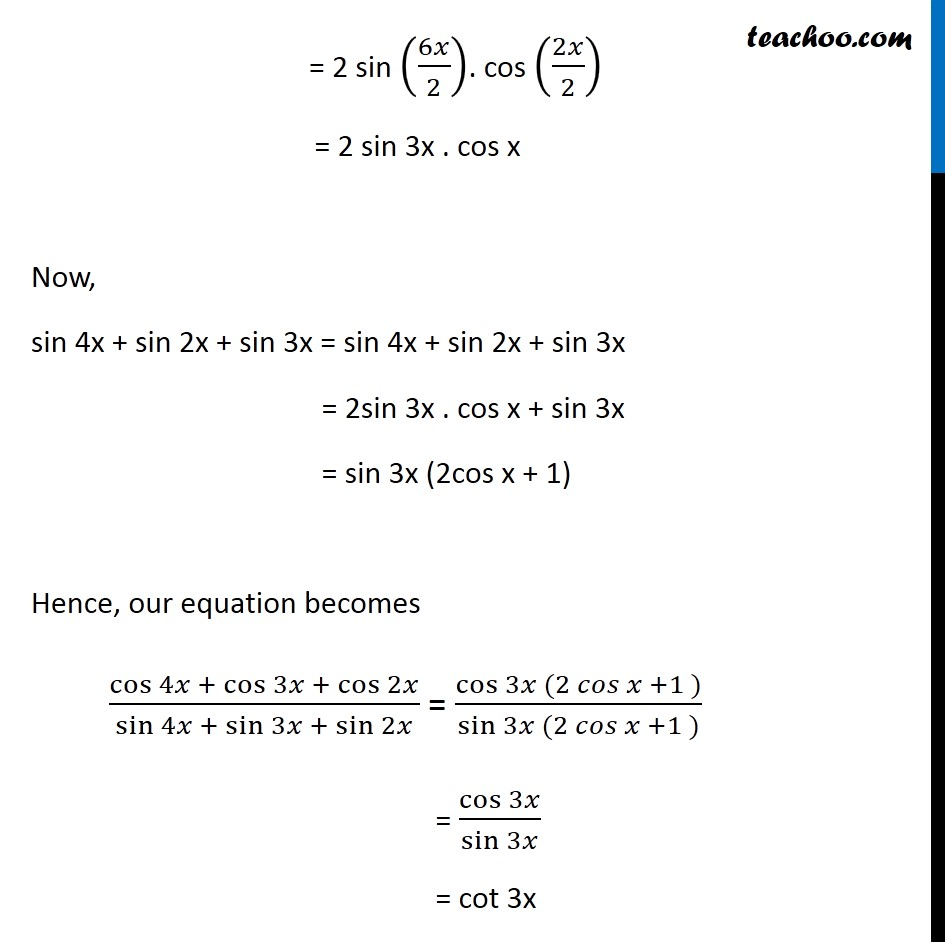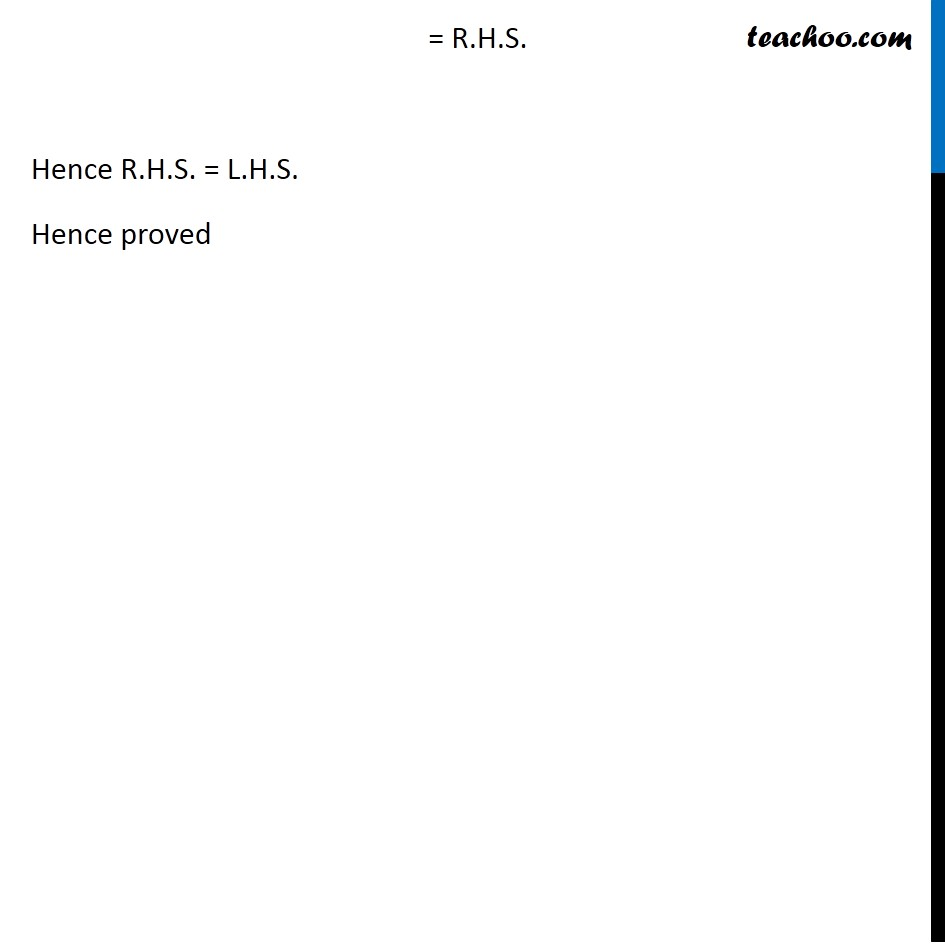Learn All Concepts of Chapter 2 Class 11 Relations and Function - FREE. Check - Trigonometry Class 11 - All Concepts1. Chapter 3 Class 11 Trigonometric Functions
2. Serial order wise
3. Ex 3.3

Transcript

Ex 3.3, 21 Prove that (cos⁡4𝑥 + cos⁡3𝑥 + cos⁡2𝑥)/(sin⁡4𝑥 + sin⁡3𝑥 + sin⁡2𝑥 ) = cot 3x Taking L.H.S Solving Numerator and Denominator separately We know that cos x + cos y = 2cos ((𝑥 + 𝑦)/2) cos ((𝑥 −𝑦)/2) Replacing x by 4x and y by 2x cos 4x + cos 2x = 2cos ((4𝑥 + 2𝑥)/2). cos ((4𝑥 − 2𝑥)/2) = 2cos (6𝑥/2). cos (2𝑥/2) = 2 cos 3x . cos x Now cos 4x + cos 2x + cos 3x = 2cos 3x . cos x + cos 3x = cos 3x (2cos x + 1) Similarly, Solving denominator sin 4x + sin 2x + sin 3x We know that sin x + sin y = 2sin ((𝑥 + 𝑦)/2) sin ((𝑥 −𝑦)/2) Replacing x by 4x and y by 2x sin 4x + sin 2x = 2 sin ((4𝑥 +2𝑥)/2). cos ((4𝑥 − 2𝑥)/2) = 2 sin (6𝑥/2). cos (2𝑥/2) = 2 sin 3x . cos x Now, sin 4x + sin 2x + sin 3x = sin 4x + sin 2x + sin 3x = 2sin 3x . cos x + sin 3x = sin 3x (2cos x + 1) Hence, our equation becomes (cos⁡4𝑥 + cos⁡3𝑥 + cos⁡2𝑥)/(sin⁡4𝑥 + sin⁡3𝑥 + sin⁡2𝑥 ) = (cos⁡3𝑥 (2 𝑐𝑜𝑠 𝑥 +1 ))/(sin⁡3𝑥 (2 𝑐𝑜𝑠 𝑥 +1 )) = cos⁡3𝑥/sin⁡3𝑥 = cot 3x = R.H.S. Hence R.H.S. = L.H.S. Hence proved

Ex 3.3